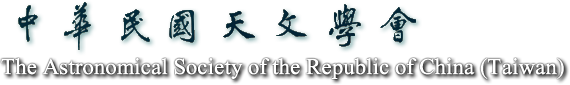2021天文年會

5月21日至23日

# 論文摘要

## Generating Kilonova Light Curves Using Autoencoder to Investigate the Properties of Compact Binary Merging Systems

### [ Oral ]

Surojit Saha(Institute of Astronomy, National Tsing Hua University )*, Laurence Datrier(Institute for Gravitational Research, School of Physics and Astronomy, University of Glasgow), Michael Williams(Institute for Gravitational Research, School of Physics and Astronomy, University of Glasgow), Albert Kong(Institute of Astronomy, National Tsing Hua University ), Ik Siong Heng(Institute for Gravitational Research, School of Physics and Astronomy, University of Glasgow), Martin Hendry(Institute for Gravitational Research, School of Physics and Astronomy, University of Glasgow), Matt Nichols(Birmingham Institute for Gravitational Wave Astronomy and School of Physics and Astronomy, University of Birmingham), Daniel Williams(Institute for Gravitational Research, School of Physics and Astronomy, University of Glasgow), Nicola De Lillo(Institute for Gravitational Research, School of Physics and Astronomy, University of Glasgow), Fergus Hayes(Institute for Gravitational Research, School of Physics and Astronomy, University of Glasgow)

The discovery of the optical counterpart, along with the gravitational waves from GW170817, of the first binary neutron star merger, opened up a new era for multi-messenger astrophysics. The optical counterpart, designated as a kilonova (KN), has immense potential to reveal the nature of compact binary merging systems. Ejecta properties from the merging system provide important information about the total binary mass, the mass ratio, system geometry, and the equation of state of the merging system. In this study, a neural network has been applied to learn the optical light curves of the KN associated with GW170817 using real data and we generate the light curves based on different ejecta properties such as lanthanide fraction, ejecta velocity and ejecta mass. For training the autoencoder, we use simulated KN light curves, where each light curve depends on ejecta mass, ejecta velocity and lanthanide fraction of the ejecta. The generated light curves using our basic autoencoder code are in good agreement with the original ones. Next, in order to verify the model built from the autoencoder, we apply denoising autoencoder to separate the noisy data from the real data. This process establishes the accuracy and robustness of the code. Following this, we develop conditional variational autoencoder (CVAE), which is for generating light curves based on the physical parameters of our choice. We have verified that CVAE can now generate light curves for any random feasible physical parameter with a satisfactory accuracy. Our CVAE is very efficient and it has a great potential to replace time consuming and resource-draining simulations. Using the CVAE, we examine detection limits associated with a given KN model. Since there are several other factors that influence KN’s light curve, we are planning to apply CVAE on different physical models and compare with real data in order to have more insights into the the physics of KN. Keywords: Kilonova, neural network, autoencoder, light curve